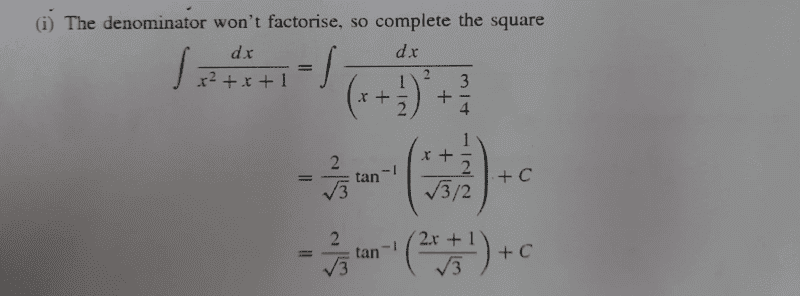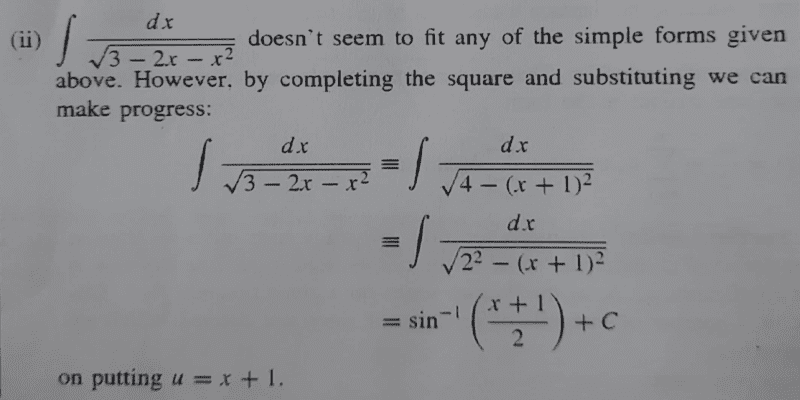# Find the indefinite integral of the given problem

• chwala

#### chwala

Gold Member
Homework Statement
see attached
Relevant Equations
##\dfrac{d}{dx} \left[\tan^{-1}x\right]= \dfrac{1}{x^2+1}##
Now the steps to solution are clear to me...My interest is on the constant that was factored out i.e ##\frac{2}{\sqrt 3}##...the steps that were followed are; They multiplied each term by ##\dfrac{2}{\sqrt 3}## to realize,

##\dfrac{2}{\sqrt 3}\int \dfrac{dx}{\left[\dfrac{2}{\sqrt 3}⋅\dfrac{2x+1}{2}\right]^2+\left[\dfrac{2}{\sqrt 3}⋅\dfrac{\sqrt 3}{2}\right]^2} ##

...

Correct? Is there a different approach guys?

Now, bringing me to my question...see problem below;Are they missing ##\dfrac{1}{2}## somewhere?? In this case we are dividing each term by ##2^2##... we ought to have;

... ##\dfrac{1}{2}\sin^{-1} \left[\frac{x+1}{2}\right] + c##

I hope i did not overlook anything...

Last edited:
•Delta2
$$\int \frac{1}{\sqrt{2^2 - (x + 1)^2}}\,dx = \int \frac{1}{2\sqrt{1 - u^2}}(2\,du)$$ with $u = (x + 1)/2$.

•Delta2 and chwala
$$\int \frac{1}{\sqrt{2^2 - (x + 1)^2}}\,dx = \int \frac{1}{2\sqrt{1 - u^2}}(2\,du)$$ with $u = (x + 1)/2$.
Thanks ...cheers man!

Aaaargh! because of the square root sign! That calls for 'change of variable' ...was wondering why...great day!